Impact Factor 3.560 | CiteScore 3.1
More on impact ›

# Frontiers in Physics## ORIGINAL RESEARCH article

Front. Phys., 24 June 2021 | https://doi.org/10.3389/fphy.2021.669459

# Towards Measuring the Maxwell–Boltzmann Distribution of a Single Heated Particle

• Molecular Nanophotonics Group, Peter Debye Institute for Soft Matter Physics, Leipzig University, Leipzig, Germany

The Maxwell–Boltzmann distribution is a hallmark of statistical physics in thermodynamic equilibrium linking the probability density of a particle’s kinetic energies to the temperature of the system that also determines its configurational fluctuations. This unique relation is lost for Hot Brownian Motion, e.g., when the Brownian particle is constantly heated to create an inhomogeneous temperature in the surrounding liquid. While the fluctuations of the particle in this case can be described with an effective temperature, it is not unique for all degrees of freedom and suggested to be different at different timescales. In this work, we report on our progress to measure the effective temperature of Hot Brownian Motion in the ballistic regime. We have constructed an optical setup to measure the displacement of a heated Brownian particle with a temporal resolution of 10 ns giving a corresponding spatial resolution of about 23 pm for a 0.92 $μm$ PMMA particle in water. Using a gold-coated polystyrene (AuPS) particle of 2.15 $μm$ diameter we determine the mean squared displacement of the particle over more than six orders of magnitude in time. Our data recovers the trends for the effective temperature at long timescales, yet shows also clear effects in the region of hydrodynamic long time tails.

## 1 Introduction

Brownian motion provides a window to microscopic dynamics. Observing the erratic motion of a colloid in a fluid delivers information on the strong interconnection of fluctuation and dissipation summarized, for example, by the simple Stokes–Einstein relation for the diffusion coefficient that is determined by temperature and viscous friction in thermal equilibrium. Breaking this equilibrium by introducing, for example, a single laser-heated particle to the solution  also breaks the fundamental assumption of equipartition and new physical descriptions are required. However, Hot Brownian Motion can be still mapped onto the well known fluctuation–dissipation relation when introducing effective quantities for temperature and viscosity . Recent theoretical studies have shown that the effective temperatures are not unique for all degrees of freedom (i.e., rotation  and translation of colloids) due to their coupling to the generated hydrodynamic flow fields . As these flow fields due to the translation and rotation of colloids in liquids also depend on the timescale of observation, the corresponding effective temperatures shall also reveal a frequency dependence as suggested in recent theoretical work . The effective configurational temperature of Hot Brownian Motion governing the diffusion coefficient at long times shall, therefore, also differ from the effective kinetic temperature that would enter a Maxwell–Boltzmann like description. Here we explore in a sophisticated experiment the short time dynamics of a heated Brownian particle. We have constructed an optical tweezers to trap and heat colloidal particles in water. We demonstrate on isothermal particles the spatial resolution of about 23 pm at a time resolution of 10 ns, which allows us to approach the ballistic regime of Brownian motion. Introducing an additional heating of the particle we explore the capabilities of the setup to provide information on the hot ballistic motion of particles for the first time.

The one-dimensional Maxwell–Boltzmann distribution (Eq. 1) was originally used to describe the velocity distribution of atoms and molecules in an ideal gas where it is assumed that there is no interaction or potential between any pair of arbitrary particles except brief collisions,

$f(v)=M2πkBTexp(−Mv22kBT)(1)$

Here the mass of the particle M and the temperature of the system T control the distribution. While this is true for gases with elastic collisions , the Maxwell–Boltzmann distribution has also been shown to hold experimentally in condensed matter with complex intermolecular interactions [11, 12]. Yet, in liquids additional hydrodynamic effects play an important role on intermediate timescales. Liquid flows that are induced by the displacement of the colloid particle lead to long living perturbations that travel a distance of the particle radius on timescales of several microseconds . This hydrodynamic memory has to be included in the Langevin equation [16, 17] to yield the equation of motion of a colloidal particle in an incompressible liquid,

$M*x¨(t)=−6πηRx˙(t)−6R2πρfη∫0t(t−t′)−1/2x¨(t′)dt′+Fth(t)−kx(t)(2)$

The effective mass $M*=Mp+Ma$ is a result of the incompressible-fluid assumption, which now also replaces the particle mass in Eq. 1. Here $Mp$ is the particle mass and $Ma=2πR3ρf/3$ the mass of the displaced fluid. η and $ρf$ are the viscosity and density of liquid. The first term on the right side of the equation resembles the Stokes friction force of a spherical particle while the second term accounts for the vorticity memory of acceleration of the liquid . The third term is the anti-correlated thermal force which is no longer Gaussian but colored owing to the fact that the vorticity generated at the surface of the particle affects the force on the sphere at a later time [11, 15, 18]. This is now captured in Eq. 3, which shows that the correlation of the force decays with a power law. Here γ is the drag coefficient and $τf=ρfR2/η$, estimating the time needed for the perturbed fluid to move to the backside of the particle,

$〈Fth(t)Fth(0)〉=−γkBTτf4πt−3/2(3)$

Finally, the last term in Eq. 2 contains the trapping force with the spring constant k.

This already complex dynamics of a Brownian particle in a dense liquid is for Hot Brownian Motion altered by a stationary inhomogeneous temperature profile taking the shape

$T(r)=T0+Pheat4πκr(4)$

where $T0$ is the ambient temperature, $Pheat$ the heating power supplied to the particle and κ the thermal conductivity of the liquid and r the distance from the particle center. This long range temperature profile is now leading to an inhomogeneous viscosity distribution in the surrounding. Both temperature increment and viscosity decrease lead to an effective diffusion coefficient of the particle

$DHBM=kBTHBM6πηHBMR(5)$

in the long-time limit, which we can use to verify our experiments on long timescales. The effective quantities $THBM$ which resembles a configurational temperature and $ηHBM$ can be calculated from the theory of Hot Brownian Motion [2, 19]. The effective temperature of Hot Brownian Motion is thereby found to be

$THBM≈T0+5ΔT/12(6)$

with $T0$ being the ambient temperature and $ΔT$ the surface temperature increment of the colloid given by the second term on the right side of Eq. 4. Estimates for the effective viscosity $ηHBM$ are reported in  for an assumed Vogel-Fulcher type temperature dependence of the viscosity of water. At shorter times, the effective temperature has required corrections with a more advanced theoretical description .

## 2 Experimental Setup

Our experimental setup is shown in Figure 1 and mainly consists of three parts. The primary part of the measurement system is for particle trapping and tracking. Light of a 1,064 nm laser (Mephisto, Coherent) is sent through a rotatable half-wave plate to adjust the IR power in the sample without modifying the driving current of the laser. A beam expander is further used to overfill the first objective (Olympus ×100/1.3NA) lens. The beam is tightly focused by this objective lens to generate a stable trapping potential  for the Brownian particles in three dimensions. A second objective is used to collect light from the trapping region that is split by a knife-edge prism and sent to a 350 MHz bandwidth balanced photodetector (Thorlabs) with a damage threshold of 20 mW. Our setup, therefore, detects the particle motion only in one dimension (e.g., the x-direction), while other dimensions may show different trapping stiffness . The analog signal is converted by a 200 MHz digitizer card and saved on the computer. The second part of the setup comprises the beam path for heating the gold nanoparticles on the polymer colloid surface at 532 nm wavelength (Sapphire, Coherent). The laser beam is passing a quarter wave plate and a polarizing beam splitter to obtain two equal-power beams with orthogonal polarization to avoid interference in the sample region. Both 532 nm beams are focused to the sample region by two lenses and two Olympus 100x/1.3NA objective lenses (where one is also used by the IR beam). The additional lenses ensure a homogeneous heating of the particles. The remaining third part (yellow) including an LED illumination and a CCD is used to check the particle trapping and particle quality.

FIGURE 1

In our experiments we use PMMA (Poly (methyl methacrylate)) polymer particles ($n=1.48$, density , microParticles) or AuPS (diameter 2.15 $μm$, effective density , microParticles) particles. The AuPS particles are polystyrene (PS) colloids coated with gold nanoparticles that have a diameter of about 8 nm covering around 10 percent of the surface of the PS particle. The gold nanoparticles are used for the PS colloid surface heating. This geometry has been used as a compromise. A larger particle mass favors a longer period of ballistic motion due to the large inertia. A larger mass, as predicted by the theory of Hot Brownian Motion , also decreases the observable effect of the heating on the effective kinetic temperature of ballistic motion. The AuPS particles thereby keep the mass of the PS particle almost unchanged, while still allowing us to heat the particle environment. All particles are suspended in water ($n=1.33$, density ). Our sample chamber is comprised of two pieces of 20 mm $×$ 20 mm microscopy cover slips with a thickness of 0.13–0.16 mm and sealed by 50-$μm$-thick tape (3 M ATG Klebstoff-Film 924) and PDMS at the boundary.

## 3 Results and Discussion

We analyze the mean squared displacement (MSD), power spectral density (PSD) and velocity distribution of the Brownian particle trapped in the optical tweezers. To decrease the statistical noise, we take 20 trajectories for each type of particle with a sampling rate of 200 MHz (10 MHz for Hot Brownian Motion) and obtain the averaged results for MSD and PSD analyses .

We first address the general performance of the setup to detect spatial displacements under isothermal conditions. In Figure 2, we show the PSD curve of the diameter PMMA particle with a trapping power $P=14.5$ mW measured at the trapping center at a temperature of 23°C. The theoretical fitting function of the PSD (see Appendix) including all hydrodynamic memory effects matches the experimental data very well. We extract a trap stiffness of about being well in the range of published results . The conversion factor reaches 5.7 mV/nm for our detection under a shot noise level of 12.0 fm/$Hz$ at high frequencies. Figure 2 also displays the power spectral density for an empty trap indicating the noise background present in the system. The PSD with the particle reaches the noise floor of the system at a frequency of around $f=7⋅106$ Hz.

FIGURE 2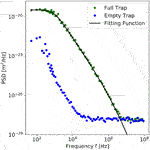FIGURE 2. Power spectral density (PSD, green dots) of a diameter PMMA particle at an ambient temperature of $T0=23$°C. The found trap stiffness corresponds to at a trapping power of $P=14.5$ mW. The black line displays the theoretical fitting function (see Appendix). The blue dots display the power spectral density of the empty trap.

We further calculate from the data the mean squared displacement. For the calculation of the MSD we take the average of every two adjacent data points to bin the position data of the balanced photodetector and subtract from the particle’s MSD the MSD calculated for the empty trap. Figure 3 displays the result of the MSD calculation together with a fit using Eq. A1, which nicely represents the measured data. The figure also displays as guides the power laws corresponding to a diffusive $(∝t)$ and a ballistic motion $(∝t)2$. The extracted trap stiffness from these experiments is determined to be and the conversion factor equals 5.7 mV/nm, which correspond well to the value obtained from the PSD. At the smallest times the MSD displacement approaches the ballistic regime, where the instantaneous momentum of the particle has not relaxed. The found momentum relaxation time of the particle is $τp=59$ ns. The time resolution of 10 ns, therefore, allows us to resolve displacements of about 23 pm, which roughly corresponds to a fifth of an atomic diameter making the detection of the particle velocity distribution more accessible . Note that this ballistic motion is different from the quasi-ballistic motion of an active system as observed in , which shows similar time dependence. There the quasi-ballistic motion observed in the time dependent MSD is likely the result of a net phoretic motion  and not related to the instantaneous momentum of the particle as in our experiments.

FIGURE 3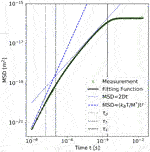FIGURE 3. Mean squared displacement determined for the diameter PMMA particle (see Figure 2 for PSD) at ambient temperature of $T0=23$°C. The green circles correspond to the experimental data after binning and subtraction while the black line displays the theoretical fitting function (see Appendix). The dotted blue line is Einstein’s prediction for diffusive motion $(∝t)$ while the dashed blue line is for ballistic motion $(∝t2)$. The vertical black lines denote the characteristic time constants of a Brownian particle in the trap. The momentum relaxation time $τp=M/(6πηR)=59$ ns, $τf=224$ ns and which is the time for the particle to move through the trap from one side to the other.

Based on this performance, we now explore the dynamics of a gold-covered polystyrene particle with a diameter for which additional heating is not applied. Figures 4, 5 show the PSD and the MSD for the trapped particle at a trapping power $P=15$ mW under a temperature of around 23.5 °C. We extract a trap stiffness from the PSD and from the MSD which implies the AuPS particle is trapped not as strongly as the PMMA particle. It is additionally reflected by a smaller corner frequency in the PSD curve of the AuPS particle. Moreover, the resulting conversion factors are 3.7 mV/nm and 3.9 mV/nm from PSD and MSD respectively under the shot noise level of 20.3 fm/$Hz$. The spatial resolution for the measurements on the AuPS particle has been determined to yield 27 pm with a temporal resolution of 40 ns which demonstrates the robustness of our particle detection.

FIGURE 4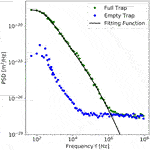FIGURE 4. Experimental power spectral density for a diameter polystyrene particle covered with gold nanoparticles (AuPS) at an ambient temperature of $T0=23.5$°C. The found trap stiffness corresponds to at a trapping power of $P=15$ mW. All symbols have the same meaning as in Figure 2.

FIGURE 5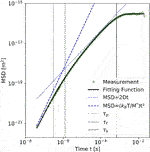FIGURE 5. Mean squared displacement for the diameter polystyrene particle covered with gold nanoparticles (AuPS) at an ambient temperature of $T0=23.5$°C. The vertical black lines denote the characteristic time constants of a Brownian particle in the trap. The momentum relaxation time $τp=M/(6πηR)=300$ ns, $τf=1251$ ns and which is the time for the particle to move through the trap from one side to the other.

Since the theoretical momentum relaxation time equals $τp=300$ ns, which is several times larger than our temporal resolution, we can use the fluctuations of the particle to calculate the probability density distribution of velocities at short times. To diminish the influence of shot noise blocking on the distribution we use binning. The bin size has been adjusted in a way such that the noise background only contributes 13% to the total kinetic energy while the minimum sampling interval is 300 ns which is still comparable with the momentum relaxation time. Figure 6 displays the obtained velocity distribution in comparison with the theoretical one. The red symbols denote the contribution of noise in the empty trap. The found experimental and theoretical root-mean-square velocities are and , respectively. Therefore, our current results with the binning to 300 ns intervals still underestimates the instantaneous velocity distribution. To reduce the binning time and the influence of noise, higher signal-to-noise ratios will be required and are currently implemented with new photodetectors. While the ultimate region of ballistic motion is not yet sufficiently well accessed, our time resolution provides access to the region of hydrodynamic long time tails, where to our knowledge no experimental data in inhomogeneous temperature fields has been available.

FIGURE 6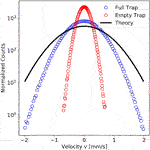FIGURE 6. Normalized velocity distribution (refer to Eq. 1 with M replaced by $M*$) obtained for the 2.15 µm diameter AuPS particle without additional heating at room temperature $T0=23.5$°C. The bin size was chosen to be 60. Blue and red circles are experimental data points for full trap and empty trap measurements. The black solid line shows the theoretical expectation for Maxwell-Boltzmann distribution at room temperature for the particle effective mass of .

To carry out measurements with a heated Brownian particle, we first measure the temperature increase near the surface of the heated AuPS particle in a separate experiment. For this purpose, we exploit the phase transition of a liquid crystal (5CB, see  for more details). The inset in Figure 7 displays the results of the measured surface temperature increases converted for when the AuPS particle is immersed in water with an incident laser at 532 nm wavelength under heating powers of 9.34, 12.66, 16.12 and 19.12 mW. The temperature increase $ΔT$ grows linearly with the heating power P as expected . Equipped with a calibration of the surface temperature we now study the dynamics of heated particles in the optical trap. We use a lower sampling rate of 10 MHz with a minimal time resolution of 100 ns for the experiment to improve the stability at long time scales.

FIGURE 7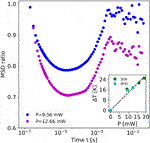FIGURE 7. Calculated ratio of the mean squared displacement for the 2.15 µm diameter AuPS particle at different heating powers with a 532 nm laser (see legend). The inset displays the surface temperature increment of the colloids as determined from a separate measurement in a liquid crystal (5CB, see text) as well as from the effective temperature of Hot Brownian Motion ($THBM$) at large time lags.

Figure 7 shows the resulting MSD ratios for a single AuPS particle trapped with 12 mW laser power (1,064 nm) at a temperature of 23.8 °C. A 532 nm laser as mentioned above with a power of 9.56 and 12.66 mW is split into two equal but perpendicularly polarized beams that are focused to a beam waist of . As the changes in the time dependence of the MSD are small upon heating, we calculate the ratio of the MSD of the particle when it is not heated to the MSDs of the particle when it is heated by the green laser . At large times, $t>1$ ms, the ratio is essentially flat and determined by the plateau of the mean squared displacement, i.e., the trapping potential. As the motion in this regime is related to the effective configuration temperature , the decreasing ratio suggests a higher effective temperature. This higher temperature is predicted by the theory of Hot Brownian Motion . We obtain the effective Hot Brownian temperatures $THBM$ from fitting the diffusive part of the MSD. Using Eq. 6 we convert the effective temperature to the surface temperature increase of the particle $ΔT$, which is displayed together with liquid crystal measurement results in the inset of Figure 7. Reasonable agreement is found suggesting that the observed trend is due to Hot Brownian Motion. Below a time lag of $t<1$ ms, much stronger changes in the MSD ratio are observed. The ratio is decreasing down to a timescale of . In this regime the Brownian motion of an isothermal particle turns from the hydrodynamic long time tails toward a diffusive motion. We suspect that the strong changes are due to the altered hydrodynamic memory of the heated liquid, though no detailed analytical model to describe the MSD in this region exists yet. At very short timescales (), the MSD ratio starts to increase, indicating smaller modifications of the particle dynamics toward a region of ballistic motion.

## 4 Conclusion

In summary, we have constructed an experimental setup to explore the short time dynamics of heated Brownian particles known as Hot Brownian Motion. With the help of isothermal liquid environments and Poly (methyl methacrylate) (PMMA) particles, we have demonstrated a spatial resolution of 23 pm with a time resolution of 10 ns with our setup. With this time resolution we are close to resolving the ballistic motion of the PMMA particle. We have further used gold-nanoparticle covered polystyrene particles to allow an optical heating of the particle surface in the trap. We observe direct indication for Hot Brownian Motion at long timescales as well as strong effects of the increased surface temperature on the hydrodynamic long-time-tails in the mean squared displacement. Our experiments demonstrate for the first time that with the help of such a setup and particles the ballistic regime of a single heated particle in a dense liquid could become accessible to studies exploring new regimes of non-equilibrium physics, for example the Maxwell–Boltzmann temperature of a heated Brownian particle.

## Data Availability Statement

The raw data supporting the conclusions of this article will be made available by the authors, without undue reservation.

## Author Contributions

FC conceived the experiment. XS and AF carried out the experiments and data analysis. FC, XS and AF wrote the manuscript.

## Funding

The authors acknowledge financial support by the German Research Foundation (Deutsche Forschungsgemeinschaft, DFG) through project number 336492136. We acknowledge support from Leipzig University for Open Access Publishing.

## Conflict of Interest

The authors declare that the research was conducted in the absence of any commercial or financial relationships that could be construed as a potential conflict of interest.

## Acknowledgments

We thank A. Kramer for helping to revise the manuscript. We thank M. Selmke for his help constructing the setup.

## References

1. Radünz R, Rings D, Kroy K, Cichos F. Hot Brownian Particles and Photothermal Correlation Spectroscopy. J Phys Chem A (2009) 113:1674–7. doi:10.1021/jp810466y

2. Rings D, Schachoff R, Selmke M, Cichos F, Kroy K. Hot Brownian Motion. Phys Rev Lett (2010) 105:090604. doi:10.1103/PhysRevLett.105.090604

3. Ruijgrok PV, Verhart NR, Zijlstra P, Tchebotareva AL, Orrit M. Brownian Fluctuations and Heating of an Optically Aligned Gold Nanorod. Phys Rev Lett (2011) 107:037401. doi:10.1103/PhysRevLett.107.037401

4. Català F, Marsà F, Montes-Usategui M, Farré A, Martín-Badosa E. Influence of Experimental Parameters on the Laser Heating of an Optical Trap. Sci Rep (2017) 7:16052. doi:10.1038/s41598-017-15904-6

5. Sevilla PR, Arita Y, Liu X, Jaque D, Dholakia K. The Temperature of an Optically Trapped, Rotating Microparticle. ACS Photon (2018) 5. doi:10.1021/acsphotonics.8b00822

6. Rodríguez-Rodríguez H, Salas G, Arias-Gonzalez JR. Heat Generation in Single Magnetic Nanoparticles under Near-Infrared Irradiation. J Phys Chem Lett (2020) 11:2182–7. doi:10.1021/acs.jpclett.0c00143

7. Chakraborty D, Gnann MV, Rings D, Glaser J, Otto F, Cichos F, et al. Generalised Einstein Relation for Hot Brownian Motion. Epl (2011) 96. doi:10.1209/0295-5075/96/60009

8. Rings D, Chakraborty D, Kroy K. Rotational Hot Brownian Motion. New J Phys (2012) 14:053012. doi:10.1088/1367-2630/14/5/053012

9. Falasco G, Gnann MV, Rings D, Kroy K. Effective Temperatures of Hot Brownian Motion. Phys Rev E Stat Nonlin Soft Matter Phys (2014) 90:032131. doi:10.1103/PhysRevE.90.032131

10. Li T, Kheifets S, Medellin D, Raizen MG. Measurement of the Instantaneous Velocity of a Brownian Particle. Science (2010) 328:1673–5. doi:10.1126/science.1189403

11. Kheifets S, Simha A, Melin K, Li T, Raizen MG. Observation of Brownian Motion in Liquids at Short Times: Instantaneous Velocity and Memory Loss. Science (2014) 343:1493–6. doi:10.1126/science.1248091

12. Mo J, Simha A, Kheifets S, Raizen MG. Testing the Maxwell-Boltzmann Distribution Using Brownian Particles. Opt Express (2015) 23:1888–93. doi:10.1364/OE.23.001888

13. Henderson S, Mitchell S, Bartlett P. Propagation of Hydrodynamic Interactions in Colloidal Suspensions. Phys Rev Lett (2002) 88:088302. doi:10.1103/PhysRevLett.88.088302

14. Lukic B, Jeney S, Tischer C, Kulik AJ, Forro L, Florin EL. Direct Observation of Nondiffusive Motion of a Brownian Particle. Phys Rev Lett (2005) 95:160601. doi:10.1103/PhysRevLett.95.160601

15. Franosch T, Grimm M, Belushkin M, Mor FM, Foffi G, Forró L, et al. Resonances Arising from Hydrodynamic Memory in Brownian Motion. Nature (2011) 478:85–8. doi:10.1038/nature10498

16. Nelson E. Dynamical Theories of Brownian Motion. Princeton University Press (1967).

17. Uhlenbeck GE, Ornstein LS. On the Theory of the Brownian Motion. Phys Rev (1930) 36. doi:10.1103/physrev.36.823

18. Li T, Raizen MG. Brownian Motion at Short Time Scales. Berlin: Ann. Phys. (2013). p. 525.

19. Rings D, Selmke M, Cichos F, Kroy K. Theory of Hot Brownian Motion. Soft Matter (2011) 7:3441-3452. doi:10.1039/c0sm00854k

20. Ashkin A. Forces of a Single-Beam Gradient Laser Trap on a Dielectric Sphere in the ray Optics Regime. Biophys J (1992) 61:569–82. doi:10.1016/S0006-3495(92)81860-X

21. Rohrbach A, Stelzer EH. Trapping Forces, Force Constants, and Potential Depths for Dielectric Spheres in the Presence of Spherical Aberrations. Appl Opt (2002) 41:2494–507. doi:10.1364/ao.41.002494

22. Grier DG. A Revolution in Optical Manipulation. Nature (2003) 424:810–816. doi:10.1038/nature01935

23. Magazzu A, Spadaro D, Donato MG, Sayed R, Messina E, D’Andrea C, et al. Optical Tweezers: a Non-destructive Tool for Soft and Biomaterial Investigations. Rend Fis Acc Lincei (2015) 26:203–218. doi:10.1007/s12210-015-0395-4

24. Rohrbach A. Stiffness of Optical Traps: Quantitative Agreement between Experiment and Electromagnetic Theory. Phys Rev Lett (2005) 95:168102. doi:10.1103/PhysRevLett.95.168102

25. Jones PH, Maragò OM, Volpe G. Optical Tweezers - Principles and Applications. Cambridge University Press (2015).

26. Flyvbjerg H, Berg-Sorensen K. Power Spectrum Analysis for Optical Tweezers. Rev Scientific Instr (2004) 75.

27. Nørrelykke SF, Flyvbjerg H. Harmonic Oscillator in Heat bath: Exact Simulation of Time-Lapse-Recorded Data and Exact Analytical Benchmark Statistics. Phys Rev E (2011) 83:041103. doi:10.1103/PhysRevE.83.041103

28. Huang R, Chavez I, Taute KM, Luki B, Jeney S, Raizen MG, et al. Direct observation of the full transition from ballistic to di_usive Brownian motion in a liquild. Nat Phys (2011) 7:576–580. doi:10.1038/nphys1953

29. Kumar S, Kumar A, Gunaseelan M, Vaippully R, Chakraborty D, Senthilselvan J, et al. Trapped in Out-Of-Equilibrium Stationary State: Hot Brownian Motion in Optically Trapped Upconverting Nanoparticles. Front Phys (2020) 8:429. doi:10.3389/fphy.2020.570842

30. Schmidt F, Magazzù A, Callegari A, Biancofiore L, Cichos F, Volpe G. Microscopic Engine Powered by Critical Demixing. Phys Rev Lett (2018) 120:068004. doi:10.1103/PhysRevLett.120.068004

31. Fränzl M, Muios-Landin S, Holubec V, Cichos F. Fully Steerable Symmetric Thermoplasmonic Microswimmers. ACS Nano (2021) 15:3434-3440. doi:10.1021/acsnano.0c10598

## Appendix

We use the following mean squared displacement of the particle for fitting, where $z1$,$z2$,$z3$,$z4$ are four roots of Eq. A2.

$〈[Δx(t)]2〉=2kBTk+2kBTM*[ez12terfc(z1t)z1(z1−z2)(z1−z3)(z1−z4)+ez22terfc(z2t)z2(z2−z1)(z2−z3)(z2−z4)+ez32terfc(z3t)z3(z3−z1)(z3−z2)(z3−z4)+ez42terfc(z4t)z4(z4−z1)(z4−z2)(z4−z3)](A1)$
$(τp+19τf)z4−τfz3+z2+1τk=0(A2)$

The power spectral density (the squared modulus of the position signal’s Fourier transform) in Eq. A3, which is normalized by the measurement time of one trajectory, is fitted with the following equation

$PSD(f)=D2π2f2×1+f2ff(fkf−f2ff−ffp−f9ff)2+(1+f2ff)2(A3)$

Here D is the diffusion coefficient and $fp,f,k$ = 1/(2π$τp,f,k$).

Keywords: Brownian motion, non-equilibrium model, frequency-dependent temperature, optical tweezers, Maxwell-Boltzmann distribution

Citation: Su X, Fischer A and Cichos F (2021) Towards Measuring the Maxwell–Boltzmann Distribution of a Single Heated Particle. Front. Phys. 9:669459. doi: 10.3389/fphy.2021.669459

Received: 18 February 2021; Accepted: 01 June 2021;
Published: 24 June 2021.

Edited by:

Ayan Banerjee, Indian Institute of Science Education and Research Kolkata, India

Reviewed by:

Basudev Roy, Indian Institute of Technology Madras, India
Raúl A. Rica, University of Granada, Spain

Copyright © 2021 Su, Fischer and Cichos. This is an open-access article distributed under the terms of the Creative Commons Attribution License (CC BY). The use, distribution or reproduction in other forums is permitted, provided the original author(s) and the copyright owner(s) are credited and that the original publication in this journal is cited, in accordance with accepted academic practice. No use, distribution or reproduction is permitted which does not comply with these terms.

*Correspondence: Frank Cichos, cichos@physik.uni-leipzig.de